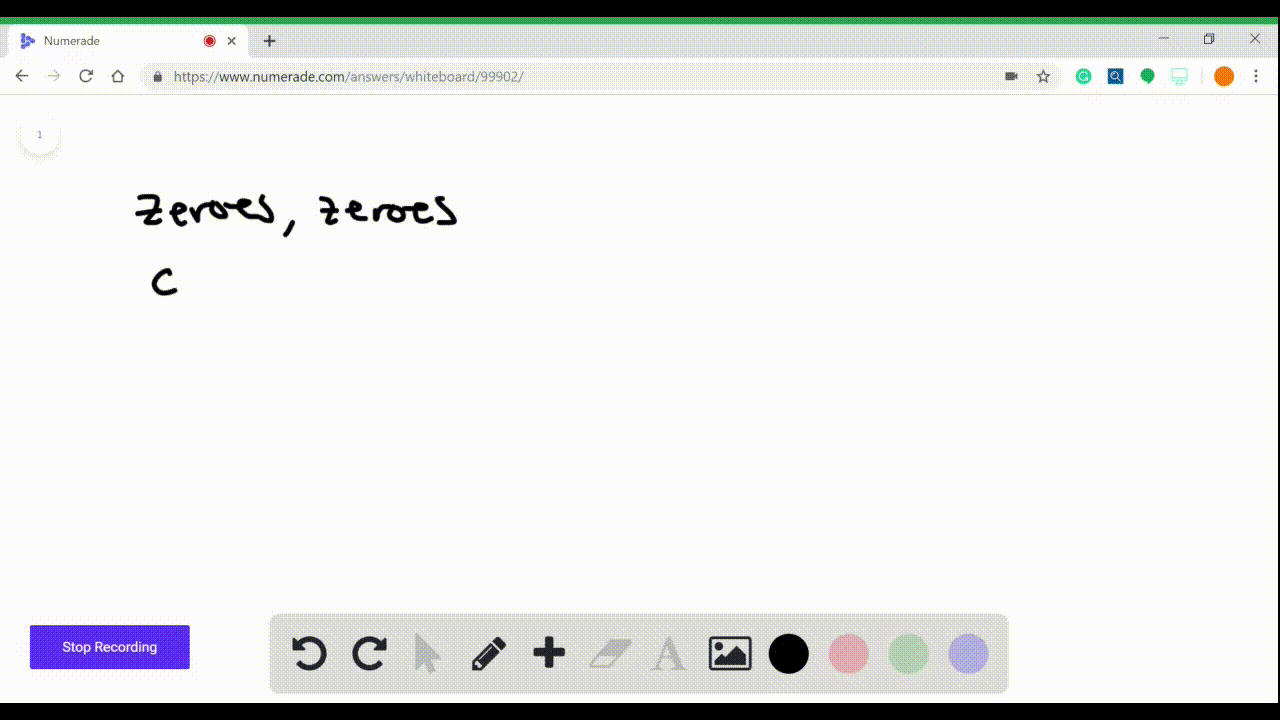Enroll in one of our FREE online STEM summer camps. Space is limited so join now!View Summer Courses### To solve a rational inequality, we factor the num…

07:10Other Schools

Need more help? Fill out this quick form to get professional live tutoring.

Get live tutoring
Problem 1

To solve a polynomial inequality, we factor the polynomial
into irreducible factors and find all the real_______polynomial. Then we find the intervals determined by the real__________sign of the polynomial on that interval. Let
$$P(x)=x(x+2)(x-1)$$
Fill in the diagram below to find the intervals on which
$P(x) \geq 0$
From the diagram above we see that $P(x) \geq 0$ on the
intervals_______and________.

## Discussion

You must be signed in to discuss.

## Video Transcript

question, and I'm not gonna write it the whole question. But it says to solve a polynomial inequality, we fact the polynomial into irreducible factors and find all the real. And there's a blank. And what we're trying to do is find the real roots. So the first blank that we're gonna fill in his roots. They were trying to find the real root to the polynomial. And then it says, Then we find the interval to turn by the rial roots sign of the polynomial on that interval. So again, this is the same blank bling and roots again, and then it gives us an example. It's his p of X is X X plus two and then x minus one, and it wants us to filling the diagram. So what they're doing is making a number line like this, and the roots are going to be minus two, which comes from this route from this factors Ari and then zero, which comes from this one. And then one is the last route, and it comes from this guy. So minus one plus one is zero. So we basically he opted science of in it. It actually asks what's the sign off a bunch of these X packs, plus two and then X minus one. And then finally, what's the sign of the whole thing together then? So, essentially, what we do is we look at a number that's less than minus two. Okay, so let's say minus three. So Axe is gonna be negative. Minus three plus two is also negative. Negative one minus three minus one is minus four. So it's negative. So there's three. Hang this over him all by three negatives. Together, we get a negative. Then I need a number between minus two and zero. So you kind of think of what I did is I plugged in minus three. That let's plug in minus one. So minus one lived in there is negative minus one plus two becomes positive minus one minus. One is negative. So that becomes positive. And then between zero and one. Let's say I put in 1/2 Okay, so X would be ah half. So it's positive we don't need to write in the actual value. We just need to write in whether it's positive or negative. So we're gonna say that's positive. Ah, half plus two is also positive and 1/2 minus. One is negative. So we get this to be negative. And then finally we take another bigger than one. So let's say two. Then that would mean that X would be too. So it's positive. X plus two would be four. Also, positive X minus one would be to minus one, which is one. So that's positive, which means the whole thing is positive. Okay, so then it says we see then from this diagram that P of X is greater than or equal to zero when it's positive. So it's on the intervals between minus two and zero. So X belongs the interval from minus 2 to 0, and then the other one is from two to infinity because that's where they're positive. This guy's positive here, and this guy's positive. So between minus two and zero on them from to infinity did that some of you that question